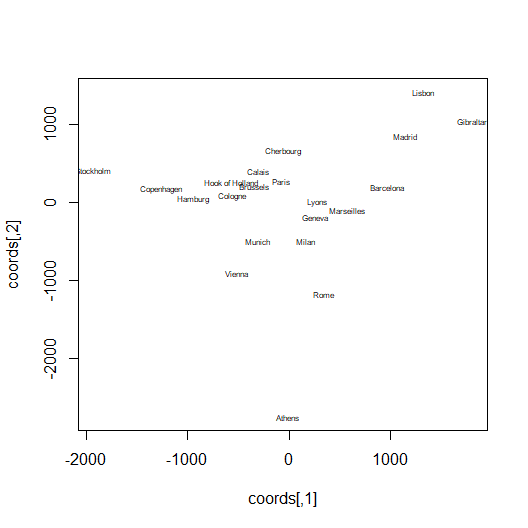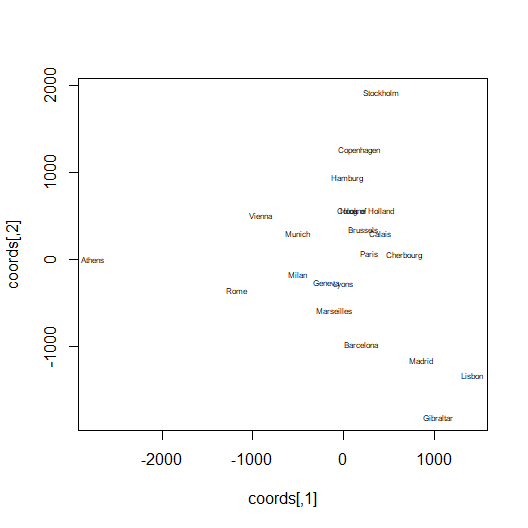# Metric MDS

#### 2019-07-10

Minimizing the Rosenbrock function is all very well, but there’s often a more complex relationship between the parameters we’re looking to optimize and what would be convenient to pass to a cost function. In many cases there are other quantities that we need to define and store somewhere for the cost function to provide a value, but which aren’t under our direct control and hence are not suitable for optimization. They just need to be available for evaluation inside fn and gr.

This vignette is less to do with the running of mize itself, and more to do with using it to solve an actual problem. It contains plenty of R code, but not a lot of it is mize-specific. Hopefully it will demonstrate how it can be used for non-trivial problems.

### Metric Multi-Dimensional Scaling

Metric multi-dimensional scaling (Metric MDS) is a way to provide a low-dimensional (usually two-dimensional) view of a high dimensional data set, although all it needs is a distance matrix to work off.

Let’s take the eurodist dataset as an example, which can be found in the datasets package. The description says:

The eurodist gives the road distances (in km) between 21 cities in Europe.

We should be able to reconstruct the relative locations of the 21 cities on a 2D plot with this information, subject to some error because roads aren’t straight between the cities and the cities themselves lie on the non-flat surface of the Earth.

### The Metric MDS Cost Function

The parameters we’re going to optimize are the 2D locations of each city. The cost function, however, is more naturally expressed as being related to the distance matrices. What we want is for the distance matrix of the output, which we’ll call $$D$$, to resemble the input distance matrix, $$R$$, as much as possible.

One obvious way to express this as a cost function is to consider the square loss:

$C = \sum_{i<j} \left(r_{ij} - d_{ij}\right)^2$ where $$r_{ij}$$ is the distance between city $$i$$ and city $$j$$ in the input distance matrix, and $$d_{ij}$$ represents the distance in the output configuration. The $$i<j$$ bit indicates that we only want to count each $$i,j$$ pair twice, because distances are symmetric. Normally, you’d probably want to divide this result by the number of pairs and then take the square root to get the units of the error as a distance and not dependent on the size of the data set, but we won’t worry about that.

As R code, the cost function can be written as:

# R and D are the input and output distance matrices, respectively
cost_fun <- function(R, D) {
diff2 <- (R - D) ^ 2
sum(diff2) * 0.5
}

It’s not good R style to use single character upper-case variables, but for matrices, we’ll get away with it just this once. I’ve also taken advantage of the vectorization of R code for matrices, rather than explicitly loop. As the calculation takes place over the entire matrix, I’ve halved the result to avoid the double counting of each distance.

Both $$R$$ and $$D$$ in the R code are of class matrix rather than the dist type that eurodist is. The matrix class is more convenient for the computations that are needed, but not as efficient in terms of storage. The eurodist data can be converted using as.matrix:

eurodist_mat <- as.matrix(eurodist)

### The Metric MDS Gradient

Writing out the cost function with respect to distances only makes it pretty straightforward. However, we need the gradient with respect to the parameters we are optimizing, which is the coordinates of each city. Indicating the (two-dimensional) vector that represents the coordintes of city $$i$$ as $$\mathbf{y_i}$$, the gradient of the cost function above is:

$\frac{\partial C}{\partial \mathbf{y_i}} = -2\sum_j \frac{r_{ij} - d_{ij}}{d_{ij}} \left( \mathbf{y_i - y_j} \right)$ where the sum $$j$$ is over all the other cities.

As R code, this is:

# R is the input distance matrix
# D is the output distance matrix
# y is a n x d matrix of output coordinates
cost_grad <- function(R, D, y) {
K <- (R - D) / (D + 1.e-10)

G <- matrix(nrow = nrow(y), ncol = ncol(y))

for (i in 1:nrow(y)) {
dyij <- sweep(-y, 2, -y[i, ])
G[i, ] <- apply(dyij * K[, i], 2, sum)
}

as.vector(t(G)) * -2
}

That loop in the middle is a bit cryptic with its sweeps and applys, but it does the job of calculating the $$\sum_j \mathbf{y_i} - \mathbf{y_j}$$ part of the gradient for each row of the gradient matrix.

### mize function and gradient

We can now write the function and gradient routines that have the interface that mize is expecting:

mmds_fn <- function(par) {
R <- as.matrix(eurodist)
y <- matrix(par, ncol = 2, byrow = TRUE)
D <- as.matrix(stats::dist(y))

cost_fun(R, D)
}
mmds_gr <- function(par) {
R <- as.matrix(eurodist)
y <- matrix(par, ncol = 2, byrow = TRUE)
D <- as.matrix(stats::dist(y))

}

These routines are not a model of efficiency. Not a problem for this small dataset, but we’ll have to revisit this below.

For now, let’s choose a starting point and get optimizing. We may as well begin from a random location:

set.seed(42)
ed0 <- rnorm(attr(eurodist, 'Size') * 2)

res_euro <- mize(ed0, list(fn = mmds_fn, gr = mmds_gr),
method = "L-BFGS", verbose = TRUE,
grad_tol = 1e-5, check_conv_every = 10)
#> 22:57:02 iter 0 f = 6.432e+08 g2 = 1.979e+05 nf = 1 ng = 1 step = 0 alpha = 0
#> 22:57:02 iter 10 f = 5.291e+07 g2 = 1.767e+04 nf = 26 ng = 26 step = 1291 alpha = 0.6507
#> 22:57:02 iter 20 f = 1.087e+07 g2 = 1.223e+04 nf = 39 ng = 39 step = 920.1 alpha = 0.4846
#> 22:57:02 iter 30 f = 3.357e+06 g2 = 129.3 nf = 49 ng = 49 step = 4.665 alpha = 0.5486
#> 22:57:02 iter 40 f = 3.356e+06 g2 = 0.2007 nf = 60 ng = 60 step = 0.03083 alpha = 1
#> 22:57:02 iter 50 f = 3.356e+06 g2 = 0.0003381 nf = 71 ng = 71 step = 2.863e-05 alpha = 1

It takes 90 iterations to converge, but we get there. Now for the moment of truth: are the cities laid out in a way we’d expect? Here’s a function that will plot the par results:

plot_mmds <- function(coords, dist, ...) {
if (class(coords) == "numeric") {
coords <- matrix(coords, ncol = 2, byrow = TRUE)
}
graphics::plot(coords, type = 'n')
graphics::text(coords[, 1], coords[, 2], labels = labels(dist), ...)
}
plot_mmds(res_euro$par, eurodist, cex = 0.5)Ah yes, Athens up in the top-right of a map of Europe, just as we expect. Ahem. In fact, because we are only optimizing distances, not absolute positions, there’s no reason that, starting from a random configuration, north will be “up” in the optimized results. Just to prove this works, let’s rotate the coordinates by 90 degrees clockwise: rot90 <- matrix(c(0, -1, 1, 0), ncol = 2) rotated <- t(rot90 %*% t(matrix(res_euro$par, ncol = 2, byrow = TRUE)))
plot_mmds(rotated, eurodist, cex = 0.5)That’s better.

### Improving the Efficiency of the Function and Gradient Routines

So the mize-powered MMDS routine is working. But it’s un-necessarily inefficient. Every time the function or gradient is calculated, we convert eurodist into a matrix. This only needs to be done once, as long as the resulting matrix is in scope inside the function. Also, if the function and gradient is calculated for the same value of par, par is converted to a distance matrix twice.

The first issue can be fixed without polluting global scope with the converted eurodist matrix by making the function and gradient closures with access to the input distance matrix. The second problem can be alleviated by adding a third item in the list passed to mize. Add a function called fg. This should calculate the cost function and gradient in one call, returning the values in a list. Routines that need to calculate the function and gradient for the same value of par will look for fg and call that, in preference to calling fn and gr separately.

Putting it all together, a function to create a suitable fg list to pass to mize is:

make_fg <- function(distmat) {
R <- as.matrix(distmat)
cost_fun <- function(R, D) {
diff2 <- (R - D) ^ 2
sum(diff2) * 0.5
}
cost_grad <- function(R, D, y) {
K <- (R - D) / (D + 1.e-10)

G <- matrix(nrow = nrow(y), ncol = ncol(y))

for (i in 1:nrow(y)) {
dyij <- sweep(-y, 2, -y[i, ])
G[i, ] <- apply(dyij * K[, i], 2, sum)
}

as.vector(t(G)) * -2
}
list(
fn = function(par) {
y <- matrix(par, ncol = 2, byrow = TRUE)
D <- as.matrix(stats::dist(y))
cost_fun(R, D)
},
gr = function(par) {
y <- matrix(par, ncol = 2, byrow = TRUE)
D <- as.matrix(stats::dist(y))
},
fg = function(par) {
y <- matrix(par, ncol = 2, byrow = TRUE)
D <- as.matrix(stats::dist(y))
list(
fn = cost_fun(R, D),
gr = cost_grad(R, D, y)
)
}
)
}

We can then run the MMDS optimization as before:

res_euro <- mize(ed0, make_fg(eurodist),
method = "L-BFGS", verbose = TRUE,
grad_tol = 1e-5, check_conv_every = 10)
#> 22:57:02 iter 0 f = 6.432e+08 g2 = 1.979e+05 nf = 1 ng = 1 step = 0 alpha = 0
#> 22:57:02 iter 10 f = 5.291e+07 g2 = 1.767e+04 nf = 26 ng = 26 step = 1291 alpha = 0.6507
#> 22:57:02 iter 20 f = 1.087e+07 g2 = 1.223e+04 nf = 39 ng = 39 step = 920.1 alpha = 0.4846
#> 22:57:02 iter 30 f = 3.357e+06 g2 = 129.3 nf = 49 ng = 49 step = 4.665 alpha = 0.5486
#> 22:57:02 iter 40 f = 3.356e+06 g2 = 0.2007 nf = 60 ng = 60 step = 0.03083 alpha = 1
#> 22:57:02 iter 50 f = 3.356e+06 g2 = 0.0003381 nf = 71 ng = 71 step = 2.863e-05 alpha = 1

The same results are achieved, but it runs a tiny bit faster, although unless you are using a very slow computer you will have to take my word for it. However, as the work done to create the data that will be used for the gradient and function calculation grows larger, you should consider making use of the item.